### Home > PC > Chapter 1 > Lesson 1.1.2 > Problem1-27

1-27.
1. Factor completely. Homework Help ✎

1. 81x3y − 12x2y

2. 3(2x + 1) + (x + 5)(2x + 1)

3. 2(3x − 7)2 + (x − 2)(3x − 7)

4. m(x + y) + (x + y)2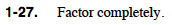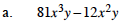Factor out the greatest common factor.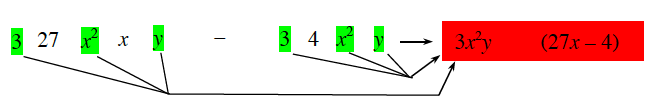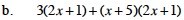Factor (2x + 1) out of each term.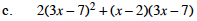Write the squared factor twice to visualize what is in common.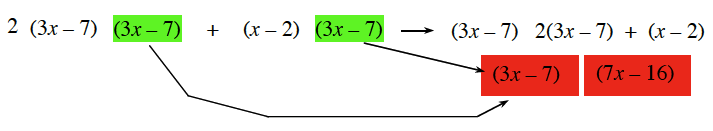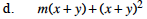Follow the same procedure in part (c).